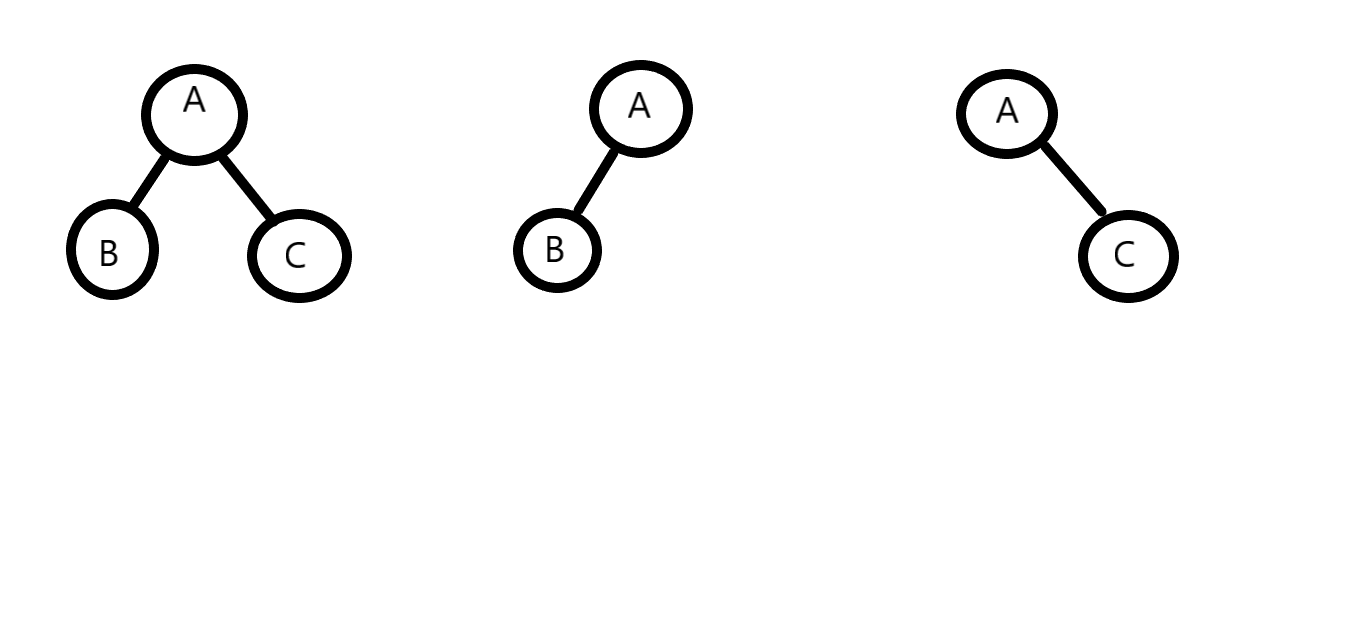# Number of balanced binary trees

Posted: 2 Jan, 2021
Difficulty: Easy

## PROBLEM STATEMENT

#### You are given an integer ‘N’, you are supposed to find the number of balanced binary trees with height ‘N’. Since the answer can be very large, return the answer modulo 10^9+7.

##### Note :
``````A binary tree is considered balanced if the difference between the left subtree’s height and the right subtree’s height is less than or equal to 1, for all non-leaf nodes.
``````

#### Example:

``````Consider N=2, there are three different binary trees.
``````##### Input Format :
``````The first line contains a single integer ‘T’ denoting the number of test cases. The test cases are as follows.

The first line of each test case contains a single integer ‘N’ denoting the height of the balanced binary tree.
``````
##### Output Format :
``````For each test case, print the number of balanced binary trees with height ‘N’ modulo 10^9+7.

Print the output of each test case on a new line.
``````
##### Note :
``````You don’t need to print anything; It has already been taken care of.
``````
##### Constraints :
``````1 <= T <= 50
1 <= N <= 10 ^ 4

Where ‘T’ denotes the number of test cases, ‘N’ denotes the height of the balanced binary tree.

Time Limit: 1 sec
``````Approach 1

For solving the problem, let us first fix the root node at level 1. Since we have fixed the root node we have N-1 levels left, now we have to count the number of ways we can create a balanced binary tree of height (N-1) at the left and the right subtree of the root node; hence we have reduced the problem from finding the number of balanced binary trees with height ‘N’ to finding the number of balanced binary trees with height ‘N-1’. This substructure property paves the way for a recursive solution.

For finding the number of balanced binary trees with height ‘N’, we will fix the root node, and then we will have the following possibilities:

1. The left subtree has a height (N-1), and the right subtree has a height (N-2).
2. The left subtree has a height (N-2), and the right subtree has a height (N-1).
3. Both the left subtree and the right subtree have a height (N-1).

The steps are as follows:

1. Let’s define a function countTree(height) which will return the number of balanced binary trees of height “height”.
2. The base case for this function will be when ‘height’ is either 0 or 1. We will have one balanced binary tree in both cases. In the case of 0, the tree will be NULL without any nodes, and for height=1, it will be a single root node.
3. Otherwise, we will recur for the left and right subtree with height “height-1” and cover the three possibilities mentioned above.
1. countTree(height-1) * countTree(height-2) {Multiplication because of independent events, i.e. our left combination doesn’t affect the right combination.}
2. countTree(height-2) * countTree(height-1) {Multiplication because of independent events, i.e. our left combination doesn’t affect the right combination.}
3. countTree(height-1) * countTree(height-1) {Multiplication because of independent events, i.e. our left combination doesn’t affect the right combination.}
4. The final answer will be the sum of the three values(Sum because of dependent events. I.e. at a time only one event can occur out of three) returned by the recursive function calls modulo 10^9+7.